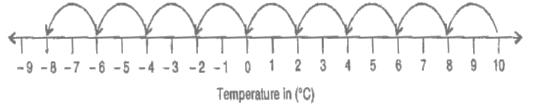# Ex.1.4 Q5 Integers Solution - NCERT Maths Class 7

Go back to  'Ex.1.4'

## Question

The temperature at $$12$$ noon was $$10^\circ \rm{c}$$  above zero. If it decreases at the rate of $$2^\circ \rm{c}$$ per hour until midnight, at what time would the temperature be $$8^\circ \rm{c}$$ below zero? What would be the temperature at mid night?Video Solution
Integers
Ex 1.4 | Question 5

## Text Solution

Steps:

The temperature at $$12$$ noon $$=10^\circ \rm{c}$$ (given)

The temperature decreases $$2^\circ \rm{c} = 1$$ hour (given)

The temperature decreases $$1^\circ \rm{c} =$$ $$1 \over 2$$hour

The temperature decreases $$18^\circ \rm{c}=\frac{1}{2} \times 18$$

(From $$10^\circ \rm{c}$$ to $$8^\circ \rm{c}$$ below zero $$=9$$ hours)

Total time

\begin{align}&= 12\, \rm{noon}+9 \,\rm{hours}\\ &= 21 \,\rm{hours}\\&= 9\, \rm{pm}\end{align}

Thus, at $$9$$ pm temperature would be $$8^\circ \rm{c}$$ below zero.

ii) The temperature at $$12$$ noon $$=10^\circ \rm{c}$$

The temperature decreases $$=2^\circ \rm{c}$$ every hour

The temperature decreases in $$12$$ hours $$=\;–2^\circ \rm{c} ×12 =24 ^\circ \rm{c}$$

At midnight, the temperature will be $$=10^\circ \rm{c}+(–24)^\circ \rm{c} =–14^\circ \rm{c}$$

Therefore, the temperature at mid night will be $$14^\circ \rm{c}$$ below $$0.$$

Learn from the best math teachers and top your exams

• Live one on one classroom and doubt clearing
• Practice worksheets in and after class for conceptual clarity
• Personalized curriculum to keep up with school